# Graph regularization for sentiment classification using synthesized graphs

## Overview

This notebook classifies movie reviews as positive or negative using the text of the review. This is an example of binary classification, an important and widely applicable kind of machine learning problem.

We will demonstrate the use of graph regularization in this notebook by building a graph from the given input. The general recipe for building a graph-regularized model using the Neural Structured Learning (NSL) framework when the input does not contain an explicit graph is as follows:

1. Create embeddings for each text sample in the input. This can be done using pre-trained models such as word2vec, Swivel, BERT etc.
2. Build a graph based on these embeddings by using a similarity metric such as the 'L2' distance, 'cosine' distance, etc. Nodes in the graph correspond to samples and edges in the graph correspond to similarity between pairs of samples.
3. Generate training data from the above synthesized graph and sample features. The resulting training data will contain neighbor features in addition to the original node features.
4. Create a neural network as a base model using the Keras sequential, functional, or subclass API.
5. Wrap the base model with the GraphRegularization wrapper class, which is provided by the NSL framework, to create a new graph Keras model. This new model will include a graph regularization loss as the regularization term in its training objective.
6. Train and evaluate the graph Keras model.

Note: We expect that it would take readers about 1 hour to go through this tutorial.

## Requirements

1. Select TensorFlow 2.x to create an interactive development environment with eager execution.
2. Install the Neural Structured Learning package.
3. Install tensorflow-hub.
``````!pip install --quiet neural-structured-learning
!pip install --quiet tensorflow-hub
``````

## Dependencies and imports

``````from __future__ import absolute_import
from __future__ import division
from __future__ import print_function
from __future__ import unicode_literals

import matplotlib.pyplot as plt
import numpy as np

import neural_structured_learning as nsl

import tensorflow as tf
tf.compat.v1.enable_v2_behavior()

import tensorflow_hub as hub

# Resets notebook state
tf.keras.backend.clear_session()

print("Version: ", tf.__version__)
print("Eager mode: ", tf.executing_eagerly())
print("Hub version: ", hub.__version__)
print("GPU is", "available" if tf.test.is_gpu_available() else "NOT AVAILABLE")
``````
```Version:  2.0.0
Eager mode:  True
Hub version:  0.6.0
GPU is NOT AVAILABLE
```

## IMDB dataset

The IMDB dataset contains the text of 50,000 movie reviews from the Internet Movie Database. These are split into 25,000 reviews for training and 25,000 reviews for testing. The training and testing sets are balanced, meaning they contain an equal number of positive and negative reviews.

In this tutorial, we will use a preprocessed version of the IMDB dataset.

The IMDB dataset comes packaged with TensorFlow. It has already been preprocessed such that the reviews (sequences of words) have been converted to sequences of integers, where each integer represents a specific word in a dictionary.

``````imdb = tf.keras.datasets.imdb
(pp_train_data, pp_train_labels), (pp_test_data, pp_test_labels) = (
``````
```Downloading data from https://storage.googleapis.com/tensorflow/tf-keras-datasets/imdb.npz
17465344/17464789 [==============================] - 0s 0us/step
```

The argument `num_words=10000` keeps the top 10,000 most frequently occurring words in the training data. The rare words are discarded to keep the size of the vocabulary manageable.

### Explore the data

Let's take a moment to understand the format of the data. The dataset comes preprocessed: each example is an array of integers representing the words of the movie review. Each label is an integer value of either 0 or 1, where 0 is a negative review, and 1 is a positive review.

``````print('Training entries: {}, labels: {}'.format(
len(pp_train_data), len(pp_train_labels)))
training_samples_count = len(pp_train_data)
``````
```Training entries: 25000, labels: 25000
```

The text of reviews have been converted to integers, where each integer represents a specific word in a dictionary. Here's what the first review looks like:

``````print(pp_train_data)
``````
```[1, 14, 22, 16, 43, 530, 973, 1622, 1385, 65, 458, 4468, 66, 3941, 4, 173, 36, 256, 5, 25, 100, 43, 838, 112, 50, 670, 2, 9, 35, 480, 284, 5, 150, 4, 172, 112, 167, 2, 336, 385, 39, 4, 172, 4536, 1111, 17, 546, 38, 13, 447, 4, 192, 50, 16, 6, 147, 2025, 19, 14, 22, 4, 1920, 4613, 469, 4, 22, 71, 87, 12, 16, 43, 530, 38, 76, 15, 13, 1247, 4, 22, 17, 515, 17, 12, 16, 626, 18, 2, 5, 62, 386, 12, 8, 316, 8, 106, 5, 4, 2223, 5244, 16, 480, 66, 3785, 33, 4, 130, 12, 16, 38, 619, 5, 25, 124, 51, 36, 135, 48, 25, 1415, 33, 6, 22, 12, 215, 28, 77, 52, 5, 14, 407, 16, 82, 2, 8, 4, 107, 117, 5952, 15, 256, 4, 2, 7, 3766, 5, 723, 36, 71, 43, 530, 476, 26, 400, 317, 46, 7, 4, 2, 1029, 13, 104, 88, 4, 381, 15, 297, 98, 32, 2071, 56, 26, 141, 6, 194, 7486, 18, 4, 226, 22, 21, 134, 476, 26, 480, 5, 144, 30, 5535, 18, 51, 36, 28, 224, 92, 25, 104, 4, 226, 65, 16, 38, 1334, 88, 12, 16, 283, 5, 16, 4472, 113, 103, 32, 15, 16, 5345, 19, 178, 32]
```

Movie reviews may be different lengths. The below code shows the number of words in the first and second reviews. Since inputs to a neural network must be the same length, we'll need to resolve this later.

``````len(pp_train_data), len(pp_train_data)
``````
`(218, 189)`

### Convert the integers back to words

It may be useful to know how to convert integers back to the corresponding text. Here, we'll create a helper function to query a dictionary object that contains the integer to string mapping:

``````def build_reverse_word_index():
# A dictionary mapping words to an integer index
word_index = imdb.get_word_index()

# The first indices are reserved
word_index = {k: (v + 3) for k, v in word_index.items()}
word_index['<START>'] = 1
word_index['<UNK>'] = 2  # unknown
word_index['<UNUSED>'] = 3
return dict((value, key) for (key, value) in word_index.items())

reverse_word_index = build_reverse_word_index()

def decode_review(text):
return ' '.join([reverse_word_index.get(i, '?') for i in text])
``````
```Downloading data from https://storage.googleapis.com/tensorflow/tf-keras-datasets/imdb_word_index.json
1646592/1641221 [==============================] - 0s 0us/step
```

Now we can use the `decode_review` function to display the text for the first review:

``````decode_review(pp_train_data)
``````
`"<START> this film was just brilliant casting location scenery story direction everyone's really suited the part they played and you could just imagine being there robert <UNK> is an amazing actor and now the same being director <UNK> father came from the same scottish island as myself so i loved the fact there was a real connection with this film the witty remarks throughout the film were great it was just brilliant so much that i bought the film as soon as it was released for <UNK> and would recommend it to everyone to watch and the fly fishing was amazing really cried at the end it was so sad and you know what they say if you cry at a film it must have been good and this definitely was also <UNK> to the two little boy's that played the <UNK> of norman and paul they were just brilliant children are often left out of the <UNK> list i think because the stars that play them all grown up are such a big profile for the whole film but these children are amazing and should be praised for what they have done don't you think the whole story was so lovely because it was true and was someone's life after all that was shared with us all"`

## Graph construction

Graph construction involves creating embeddings for text samples and then using a similarity function to compare the embeddings.

Before proceeding further, we first create a directory to store artifacts created by this tutorial.

``````!mkdir -p /tmp/imdb
``````

### Create sample embeddings

We will use pretrained Swivel embeddings to create embeddings in the `tf.train.Example` format for each sample in the input. We will store the resulting embeddings in the `TFRecord` format along with an additional feature that represents the ID of each sample. This is important and will allow us match sample embeddings with corresponding nodes in the graph later.

``````# This is necessary because hub.KerasLayer assumes tensor hashability, which
# is not supported in eager mode.
tf.compat.v1.disable_tensor_equality()

hub_layer = hub.KerasLayer(
pretrained_embedding, input_shape=[], dtype=tf.string, trainable=True)
``````
``````def _int64_feature(value):
"""Returns int64 tf.train.Feature."""
return tf.train.Feature(int64_list=tf.train.Int64List(value=value.tolist()))

def _bytes_feature(value):
"""Returns bytes tf.train.Feature."""
return tf.train.Feature(
bytes_list=tf.train.BytesList(value=[value.encode('utf-8')]))

def _float_feature(value):
"""Returns float tf.train.Feature."""
return tf.train.Feature(float_list=tf.train.FloatList(value=value.tolist()))

def create_embedding_example(word_vector, record_id):
"""Create tf.Example containing the sample's embedding and its ID."""

text = decode_review(word_vector)

# Shape = [batch_size,].
sentence_embedding = hub_layer(tf.reshape(text, shape=[-1,]))

# Flatten the sentence embedding back to 1-D.
sentence_embedding = tf.reshape(sentence_embedding, shape=[-1])

features = {
'id': _bytes_feature(str(record_id)),
'embedding': _float_feature(sentence_embedding.numpy())
}
return tf.train.Example(features=tf.train.Features(feature=features))

def create_embeddings(word_vectors, output_path, starting_record_id):
record_id = int(starting_record_id)
with tf.io.TFRecordWriter(output_path) as writer:
for word_vector in word_vectors:
example = create_embedding_example(word_vector, record_id)
record_id = record_id + 1
writer.write(example.SerializeToString())
return record_id

# Persist TF.Example features containing embeddings for training data in
# TFRecord format.
create_embeddings(pp_train_data, '/tmp/imdb/embeddings.tfr', 0)
``````
`25000`

### Build a graph

Now that we have the sample embeddings, we will use them to build a similarity graph, i.e, nodes in this graph will correspond to samples and edges in this graph will correspond to similarity between pairs of nodes.

Neural Structured Learning provides a graph building library to build a graph based on sample embeddings. It uses cosine similarity as the similarity measure to compare embeddings and build edges between them. It also allows us to specify a similarity threshold, which can be used to discard dissimilar edges from the final graph. In this example, using 0.99 as the similarity threshold, we end up with a graph that has 445,327 bi-directional edges.

``````nsl.tools.build_graph(['/tmp/imdb/embeddings.tfr'],
'/tmp/imdb/graph_99.tsv',
similarity_threshold=0.99)
``````

Note: Graph quality and by extension, embedding quality, are very important for graph regularization. While we have used Swivel embeddings in this notebook, using BERT embeddings for instance, will likely capture review semantics more accurately. We encourage users to use embeddings of their choice and as appropriate to their needs.

## Sample features

We create sample features for our problem using the `tf.train.Example` format and persist them in the `TFRecord` format. Each sample will include the following three features:

1. id: The node ID of the sample.
2. words: An int64 list containing word IDs.
3. label: A singleton int64 identifying the target class of the review.
``````def create_example(word_vector, label, record_id):
"""Create tf.Example containing the sample's word vector, label, and ID."""
features = {
'id': _bytes_feature(str(record_id)),
'words': _int64_feature(np.asarray(word_vector)),
'label': _int64_feature(np.asarray([label])),
}
return tf.train.Example(features=tf.train.Features(feature=features))

def create_records(word_vectors, labels, record_path, starting_record_id):
record_id = int(starting_record_id)
with tf.io.TFRecordWriter(record_path) as writer:
for word_vector, label in zip(word_vectors, labels):
example = create_example(word_vector, label, record_id)
record_id = record_id + 1
writer.write(example.SerializeToString())
return record_id

# Persist TF.Example features (word vectors and labels) for training and test
# data in TFRecord format.
next_record_id = create_records(pp_train_data, pp_train_labels,
'/tmp/imdb/train_data.tfr', 0)
create_records(pp_test_data, pp_test_labels, '/tmp/imdb/test_data.tfr',
next_record_id)
``````
`50000`

## Augment training data with graph neighbors

Since we have the sample features and the synthesized graph, we can generate the augmented training data for Neural Structured Learning. The NSL framework provides a library to combine the graph and the sample features to produce the final training data for graph regularization. The resulting training data will include original sample features as well as features of their corresponding neighbors.

In this tutorial, we consider undirected edges and use a maximum of 3 neighbors per sample to augment training data with graph neighbors.

``````nsl.tools.pack_nbrs(
'/tmp/imdb/train_data.tfr',
'',
'/tmp/imdb/graph_99.tsv',
'/tmp/imdb/nsl_train_data.tfr',
max_nbrs=3)
``````

## Base model

We are now ready to build a base model without graph regularization. In order to build this model, we can either use embeddings that were used in building the graph, or we can learn new embeddings jointly along with the classification task. For the purpose of this notebook, we will do the latter.

### Global variables

``````NBR_FEATURE_PREFIX = 'NL_nbr_'
NBR_WEIGHT_SUFFIX = '_weight'
``````

### Hyperparameters

We will use an instance of `HParams` to inclue various hyperparameters and constants used for training and evaluation. We briefly describe each of them below:

• num_classes: There are 2 classes -- positive and negative.

• max_seq_length: This is the maximum number of words considered from each movie review in this example.

• vocab_size: This is the size of the vocabulary considered for this example.

• distance_type: This is the distance metric used to regularize the sample with its neighbors.

• graph_regularization_multiplier: This controls the relative weight of the graph regularization term in the overall loss function.

• num_neighbors: The number of neighbors used for graph regularization. This value has to be less than or equal to the `max_nbrs` argument used above when invoking `nsl.tools.pack_nbrs`.

• num_fc_units: The number of units in the fully connected layer of the neural network.

• train_epochs: The number of training epochs.

• batch_size: Batch size used for training and evaluation.

• eval_steps: The number of batches to process before deeming evaluation is complete. If set to `None`, all instances in the test set are evaluated.

``````class HParams(object):
"""Hyperparameters used for training."""
def __init__(self):
### dataset parameters
self.num_classes = 2
self.max_seq_length = 256
self.vocab_size = 10000
### neural graph learning parameters
self.distance_type = nsl.configs.DistanceType.L2
self.graph_regularization_multiplier = 0.1
self.num_neighbors = 2
### model architecture
self.num_embedding_dims = 16
self.num_lstm_dims = 64
self.num_fc_units = 64
### training parameters
self.train_epochs = 10
self.batch_size = 128
### eval parameters
self.eval_steps = None  # All instances in the test set are evaluated.

HPARAMS = HParams()
``````

### Prepare the data

The reviews—the arrays of integers—must be converted to tensors before being fed into the neural network. This conversion can be done a couple of ways:

• Convert the arrays into vectors of `0`s and `1`s indicating word occurrence, similar to a one-hot encoding. For example, the sequence `[3, 5]` would become a `10000`-dimensional vector that is all zeros except for indices `3` and `5`, which are ones. Then, make this the first layer in our network—a `Dense` layer—that can handle floating point vector data. This approach is memory intensive, though, requiring a `num_words * num_reviews` size matrix.

• Alternatively, we can pad the arrays so they all have the same length, then create an integer tensor of shape `max_length * num_reviews`. We can use an embedding layer capable of handling this shape as the first layer in our network.

In this tutorial, we will use the second approach.

Since the movie reviews must be the same length, we will use the `pad_sequence` function defined below to standardize the lengths.

``````def pad_sequence(sequence, max_seq_length):
"""Pads the input sequence (a `tf.SparseTensor`) to `max_seq_length`."""
pad_size = tf.maximum(, max_seq_length - tf.shape(sequence))
[sequence.values,
axis=0)
# The input sequence may be larger than max_seq_length. Truncate down if
# necessary.

def parse_example(example_proto):
"""Extracts relevant fields from the `example_proto`.

Args:
example_proto: An instance of `tf.train.Example`.

Returns:
A pair whose first value is a dictionary containing relevant features
and whose second value contains the ground truth labels.
"""
# The 'words' feature is a variable length word ID vector.
feature_spec = {
'words': tf.io.VarLenFeature(tf.int64),
'label': tf.io.FixedLenFeature((), tf.int64, default_value=-1),
}
# We also extract corresponding neighbor features in a similar manner to
# the features above.
for i in range(HPARAMS.num_neighbors):
nbr_feature_key = '{}{}_{}'.format(NBR_FEATURE_PREFIX, i, 'words')
nbr_weight_key = '{}{}{}'.format(NBR_FEATURE_PREFIX, i, NBR_WEIGHT_SUFFIX)
feature_spec[nbr_feature_key] = tf.io.VarLenFeature(tf.int64)

# We assign a default value of 0.0 for the neighbor weight so that
# graph regularization is done on samples based on their exact number
# of neighbors. In other words, non-existent neighbors are discounted.
feature_spec[nbr_weight_key] = tf.io.FixedLenFeature(
, tf.float32, default_value=tf.constant([0.0]))

features = tf.io.parse_single_example(example_proto, feature_spec)

# Since the 'words' feature is a variable length word vector, we pad it to a
# constant maximum length based on HPARAMS.max_seq_length
for i in range(HPARAMS.num_neighbors):
nbr_feature_key = '{}{}_{}'.format(NBR_FEATURE_PREFIX, i, 'words')
HPARAMS.max_seq_length)

labels = features.pop('label')
return features, labels

def make_dataset(file_path, training=False):
"""Creates a `tf.data.TFRecordDataset`.

Args:
file_path: Name of the file in the `.tfrecord` format containing
`tf.train.Example` objects.
training: Boolean indicating if we are in training mode.

Returns:
An instance of `tf.data.TFRecordDataset` containing the `tf.train.Example`
objects.
"""
dataset = tf.data.TFRecordDataset([file_path])
if training:
dataset = dataset.shuffle(10000)
dataset = dataset.map(parse_example)
dataset = dataset.batch(HPARAMS.batch_size)
return dataset

train_dataset = make_dataset('/tmp/imdb/nsl_train_data.tfr', True)
test_dataset = make_dataset('/tmp/imdb/test_data.tfr')
``````

### Build the model

A neural network is created by stacking layers—this requires two main architectural decisions:

• How many layers to use in the model?
• How many hidden units to use for each layer?

In this example, the input data consists of an array of word-indices. The labels to predict are either 0 or 1.

We will use a bi-directional LSTM as our base model in this tutorial.

``````# This function exists as an alternative to the bi-LSTM model used in this
# notebook.
def make_feed_forward_model():
"""Builds a simple 2 layer feed forward neural network."""
inputs = tf.keras.Input(
shape=(HPARAMS.max_seq_length,), dtype='int64', name='words')
embedding_layer = tf.keras.layers.Embedding(HPARAMS.vocab_size, 16)(inputs)
pooling_layer = tf.keras.layers.GlobalAveragePooling1D()(embedding_layer)
dense_layer = tf.keras.layers.Dense(16, activation='relu')(pooling_layer)
outputs = tf.keras.layers.Dense(1, activation='sigmoid')(dense_layer)
return tf.keras.Model(inputs=inputs, outputs=outputs)

def make_bilstm_model():
"""Builds a bi-directional LSTM model."""
inputs = tf.keras.Input(
shape=(HPARAMS.max_seq_length,), dtype='int64', name='words')
embedding_layer = tf.keras.layers.Embedding(HPARAMS.vocab_size,
HPARAMS.num_embedding_dims)(
inputs)
lstm_layer = tf.keras.layers.Bidirectional(
tf.keras.layers.LSTM(HPARAMS.num_lstm_dims))(
embedding_layer)
dense_layer = tf.keras.layers.Dense(
HPARAMS.num_fc_units, activation='relu')(
lstm_layer)
outputs = tf.keras.layers.Dense(1, activation='sigmoid')(dense_layer)
return tf.keras.Model(inputs=inputs, outputs=outputs)

# Feel free to use an architecture of your choice.
model = make_bilstm_model()
model.summary()
``````
```Model: "model"
_________________________________________________________________
Layer (type)                 Output Shape              Param #
=================================================================
words (InputLayer)           [(None, 256)]             0
_________________________________________________________________
embedding (Embedding)        (None, 256, 16)           160000
_________________________________________________________________
bidirectional (Bidirectional (None, 128)               41472
_________________________________________________________________
dense (Dense)                (None, 64)                8256
_________________________________________________________________
dense_1 (Dense)              (None, 1)                 65
=================================================================
Total params: 209,793
Trainable params: 209,793
Non-trainable params: 0
_________________________________________________________________
```

The layers are effectively stacked sequentially to build the classifier:

1. The first layer is an `Input` layer which takes the integer-encoded vocabulary.
2. The next layer is an `Embedding` layer, which takes the integer-encoded vocabulary and looks up the embedding vector for each word-index. These vectors are learned as the model trains. The vectors add a dimension to the output array. The resulting dimensions are: `(batch, sequence, embedding)`.
3. Next, a bidirectional LSTM layer returns a fixed-length output vector for each example.
4. This fixed-length output vector is piped through a fully-connected (`Dense`) layer with 64 hidden units.
5. The last layer is densely connected with a single output node. Using the `sigmoid` activation function, this value is a float between 0 and 1, representing a probability, or confidence level.

### Hidden units

The above model has two intermediate or "hidden" layers, between the input and output, and excluding the `Embedding` layer. The number of outputs (units, nodes, or neurons) is the dimension of the representational space for the layer. In other words, the amount of freedom the network is allowed when learning an internal representation.

If a model has more hidden units (a higher-dimensional representation space), and/or more layers, then the network can learn more complex representations. However, it makes the network more computationally expensive and may lead to learning unwanted patterns—patterns that improve performance on training data but not on the test data. This is called overfitting.

### Loss function and optimizer

A model needs a loss function and an optimizer for training. Since this is a binary classification problem and the model outputs a probability (a single-unit layer with a sigmoid activation), we'll use the `binary_crossentropy` loss function.

``````model.compile(
``````

### Create a validation set

When training, we want to check the accuracy of the model on data it hasn't seen before. Create a validation set by setting apart a fraction of the original training data. (Why not use the testing set now? Our goal is to develop and tune our model using only the training data, then use the test data just once to evaluate our accuracy).

In this tutorial, we take roughly 10% of the initial training samples (10% of 25000) as labeled data for training and the remaining as validation data. Since the initial train/test split was 50/50 (25000 samples each), the effective train/validation/test split we now have is 5/45/50.

Note that 'train_dataset' has already been batched and shuffled.

``````validation_fraction = 0.9
validation_size = int(validation_fraction *
int(training_samples_count / HPARAMS.batch_size))
print(validation_size)
validation_dataset = train_dataset.take(validation_size)
train_dataset = train_dataset.skip(validation_size)
``````
```175
```

### Train the model

Train the model in mini-batches. While training, monitor the model's loss and accuracy on the validation set:

``````history = model.fit(
train_dataset,
validation_data=validation_dataset,
epochs=HPARAMS.train_epochs,
verbose=1)
``````
```Epoch 1/10
21/21 [==============================] - 45s 2s/step - loss: 0.6933 - accuracy: 0.4958 - val_loss: 0.0000e+00 - val_accuracy: 0.0000e+00
Epoch 2/10
21/21 [==============================] - 41s 2s/step - loss: 0.6926 - accuracy: 0.5142 - val_loss: 0.0000e+00 - val_accuracy: 0.0000e+00
Epoch 3/10
21/21 [==============================] - 41s 2s/step - loss: 0.6801 - accuracy: 0.6319 - val_loss: 0.0000e+00 - val_accuracy: 0.0000e+00
Epoch 4/10
21/21 [==============================] - 40s 2s/step - loss: 0.6597 - accuracy: 0.6373 - val_loss: 0.0000e+00 - val_accuracy: 0.0000e+00
Epoch 5/10
21/21 [==============================] - 41s 2s/step - loss: 0.5069 - accuracy: 0.7615 - val_loss: 0.0000e+00 - val_accuracy: 0.0000e+00
Epoch 6/10
21/21 [==============================] - 40s 2s/step - loss: 0.4001 - accuracy: 0.8277 - val_loss: 0.0000e+00 - val_accuracy: 0.0000e+00
Epoch 7/10
21/21 [==============================] - 41s 2s/step - loss: 0.3650 - accuracy: 0.8481 - val_loss: 0.0000e+00 - val_accuracy: 0.0000e+00
Epoch 8/10
21/21 [==============================] - 41s 2s/step - loss: 0.3079 - accuracy: 0.8796 - val_loss: 0.0000e+00 - val_accuracy: 0.0000e+00
Epoch 9/10
21/21 [==============================] - 40s 2s/step - loss: 0.3109 - accuracy: 0.8819 - val_loss: 0.0000e+00 - val_accuracy: 0.0000e+00
Epoch 10/10
21/21 [==============================] - 41s 2s/step - loss: 0.2839 - accuracy: 0.8912 - val_loss: 0.0000e+00 - val_accuracy: 0.0000e+00
```

### Evaluate the model

Now, let's see how the model performs. Two values will be returned. Loss (a number which represents our error, lower values are better), and accuracy.

``````results = model.evaluate(test_dataset, steps=HPARAMS.eval_steps)
print(results)
``````
```196/196 [==============================] - 28s 144ms/step - loss: 0.3815 - accuracy: 0.8442
[0.38148618154987995, 0.8442]
```

### Create a graph of accuracy/loss over time

`model.fit()` returns a `History` object that contains a dictionary with everything that happened during training:

``````history_dict = history.history
history_dict.keys()
``````
`dict_keys(['loss', 'val_loss', 'accuracy', 'val_accuracy'])`

There are four entries: one for each monitored metric during training and validation. We can use these to plot the training and validation loss for comparison, as well as the training and validation accuracy:

``````acc = history_dict['accuracy']
val_acc = history_dict['val_accuracy']
loss = history_dict['loss']
val_loss = history_dict['val_loss']

epochs = range(1, len(acc) + 1)

# "-r^" is for solid red line with triangle markers.
plt.plot(epochs, loss, '-r^', label='Training loss')
# "-b0" is for solid blue line with circle markers.
plt.plot(epochs, val_loss, '-bo', label='Validation loss')
plt.title('Training and validation loss')
plt.xlabel('Epochs')
plt.ylabel('Loss')
plt.legend(loc='best')

plt.show()
``````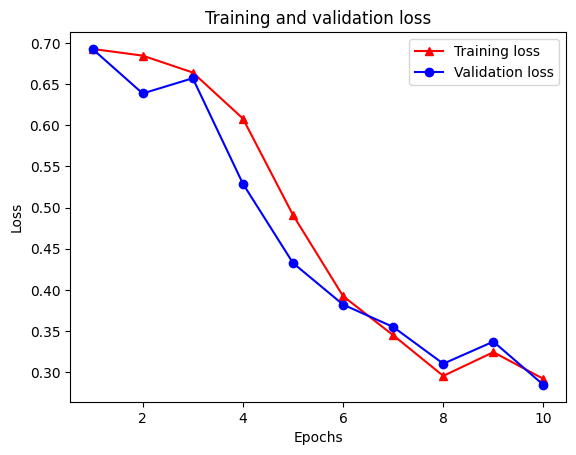``````plt.clf()   # clear figure

plt.plot(epochs, acc, '-r^', label='Training acc')
plt.plot(epochs, val_acc, '-bo', label='Validation acc')
plt.title('Training and validation accuracy')
plt.xlabel('Epochs')
plt.ylabel('Accuracy')
plt.legend(loc='best')

plt.show()
``````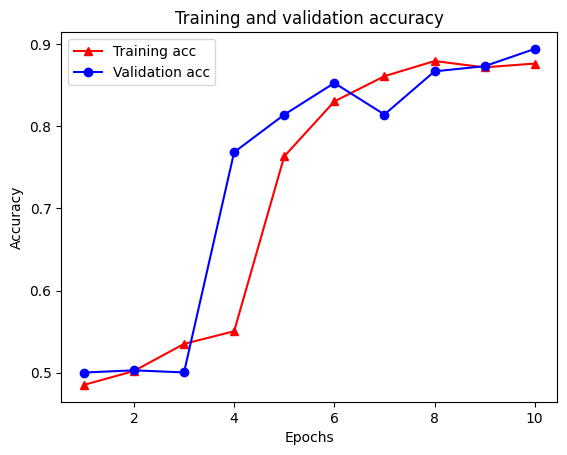Notice the training loss decreases with each epoch and the training accuracy increases with each epoch. This is expected when using a gradient descent optimization—it should minimize the desired quantity on every iteration.

## Graph regularization

We are now ready to try graph regularization using the base model that we built above. We will use the `GraphRegularization` wrapper class provided by the Neural Structured Learning framework to wrap the base (bi-LSTM) model to include graph regularization. The rest of the steps for training and evaluating the graph-regularized model are similar to that of the base model.

### Create graph-regularized model

To assess the incremental benefit of graph regularization, we will create a new base model instance. This is because `model` has already been trained for a few iterations, and reusing this trained model to create a graph-regularized model will not be a fair comparison for `model`.

``````# Build a new base LSTM model.
base_reg_model = make_bilstm_model()
``````
``````# Wrap the base model with graph regularization.
graph_reg_config = nsl.configs.make_graph_reg_config(
max_neighbors=HPARAMS.num_neighbors,
multiplier=HPARAMS.graph_regularization_multiplier,
distance_type=HPARAMS.distance_type,
sum_over_axis=-1)
graph_reg_model = nsl.keras.GraphRegularization(base_reg_model,
graph_reg_config)
graph_reg_model.compile(
``````

### Train the model

``````graph_reg_history = graph_reg_model.fit(
train_dataset,
validation_data=validation_dataset,
epochs=HPARAMS.train_epochs,
verbose=1)
``````
```Epoch 1/10

/tmpfs/src/tf_docs_env/lib/python3.5/site-packages/tensorflow_core/python/framework/indexed_slices.py:424: UserWarning: Converting sparse IndexedSlices to a dense Tensor of unknown shape. This may consume a large amount of memory.
"Converting sparse IndexedSlices to a dense Tensor of unknown shape. "
/tmpfs/src/tf_docs_env/lib/python3.5/site-packages/tensorflow_core/python/framework/indexed_slices.py:424: UserWarning: Converting sparse IndexedSlices to a dense Tensor of unknown shape. This may consume a large amount of memory.
"Converting sparse IndexedSlices to a dense Tensor of unknown shape. "

21/21 [==============================] - 69s 3s/step - loss: 0.6933 - accuracy: 0.5088 - graph_loss: 8.4686e-06 - val_loss: 0.0000e+00 - val_accuracy: 0.0000e+00 - val_graph_loss: 0.0000e+00
Epoch 2/10
21/21 [==============================] - 58s 3s/step - loss: 0.6923 - accuracy: 0.5588 - graph_loss: 7.0241e-04 - val_loss: 0.0000e+00 - val_accuracy: 0.0000e+00 - val_graph_loss: 0.0000e+00
Epoch 3/10
21/21 [==============================] - 58s 3s/step - loss: 0.6739 - accuracy: 0.6073 - graph_loss: 0.0010 - val_loss: 0.0000e+00 - val_accuracy: 0.0000e+00 - val_graph_loss: 0.0000e+00
Epoch 4/10
21/21 [==============================] - 57s 3s/step - loss: 0.6175 - accuracy: 0.6877 - graph_loss: 0.0321 - val_loss: 0.0000e+00 - val_accuracy: 0.0000e+00 - val_graph_loss: 0.0000e+00
Epoch 5/10
21/21 [==============================] - 58s 3s/step - loss: 0.5287 - accuracy: 0.7462 - graph_loss: 0.0464 - val_loss: 0.0000e+00 - val_accuracy: 0.0000e+00 - val_graph_loss: 0.0000e+00
Epoch 6/10
21/21 [==============================] - 57s 3s/step - loss: 0.4341 - accuracy: 0.8177 - graph_loss: 0.1333 - val_loss: 0.0000e+00 - val_accuracy: 0.0000e+00 - val_graph_loss: 0.0000e+00
Epoch 7/10
21/21 [==============================] - 57s 3s/step - loss: 0.3772 - accuracy: 0.8477 - graph_loss: 0.1672 - val_loss: 0.0000e+00 - val_accuracy: 0.0000e+00 - val_graph_loss: 0.0000e+00
Epoch 8/10
21/21 [==============================] - 57s 3s/step - loss: 0.3306 - accuracy: 0.8788 - graph_loss: 0.2073 - val_loss: 0.0000e+00 - val_accuracy: 0.0000e+00 - val_graph_loss: 0.0000e+00
Epoch 9/10
21/21 [==============================] - 57s 3s/step - loss: 0.3174 - accuracy: 0.8858 - graph_loss: 0.2277 - val_loss: 0.0000e+00 - val_accuracy: 0.0000e+00 - val_graph_loss: 0.0000e+00
Epoch 10/10
21/21 [==============================] - 58s 3s/step - loss: 0.2657 - accuracy: 0.9131 - graph_loss: 0.2316 - val_loss: 0.0000e+00 - val_accuracy: 0.0000e+00 - val_graph_loss: 0.0000e+00
```

### Evaluate the model

``````graph_reg_results = graph_reg_model.evaluate(test_dataset, steps=HPARAMS.eval_steps)
print(graph_reg_results)
``````
```196/196 [==============================] - 28s 145ms/step - loss: 0.3580 - accuracy: 0.8514 - graph_loss: 0.0000e+00
[0.35800924441035914, 0.85136, 0.0]
```

### Create a graph of accuracy/loss over time

``````graph_reg_history_dict = graph_reg_history.history
graph_reg_history_dict.keys()
``````
`dict_keys(['graph_loss', 'accuracy', 'val_loss', 'loss', 'val_graph_loss', 'val_accuracy'])`

There are six entries: one for each monitored metric -- loss, graph loss, and accuracy -- during training and validation. We can use these to plot the training, graph, and validation losses for comparison, as well as the training and validation accuracy. Note that the graph loss is only computed during training; so its value will be 0 during validation.

``````acc = graph_reg_history_dict['accuracy']
val_acc = graph_reg_history_dict['val_accuracy']
loss = graph_reg_history_dict['loss']
graph_loss = graph_reg_history_dict['graph_loss']
val_loss = graph_reg_history_dict['val_loss']
val_graph_loss = graph_reg_history_dict['val_graph_loss']

epochs = range(1, len(acc) + 1)

plt.clf()   # clear figure

# "-r^" is for solid red line with triangle markers.
plt.plot(epochs, loss, '-r^', label='Training loss')
# "-gD" is for solid green line with diamond markers.
plt.plot(epochs, graph_loss, '-gD', label='Training graph loss')
# "-b0" is for solid blue line with circle markers.
plt.plot(epochs, val_loss, '-bo', label='Validation loss')
# "-ms" is for solid magenta line with square markers.
plt.plot(epochs, val_graph_loss, '-ms', label='Validation graph loss')
plt.title('Training and validation loss')
plt.xlabel('Epochs')
plt.ylabel('Loss')
plt.legend(loc='best')

plt.show()
``````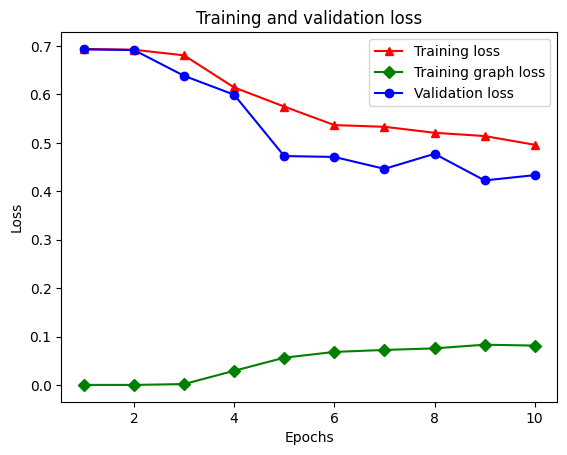``````plt.clf()   # clear figure

plt.plot(epochs, acc, '-r^', label='Training acc')
plt.plot(epochs, val_acc, '-bo', label='Validation acc')
plt.title('Training and validation accuracy')
plt.xlabel('Epochs')
plt.ylabel('Accuracy')
plt.legend(loc='best')

plt.show()
``````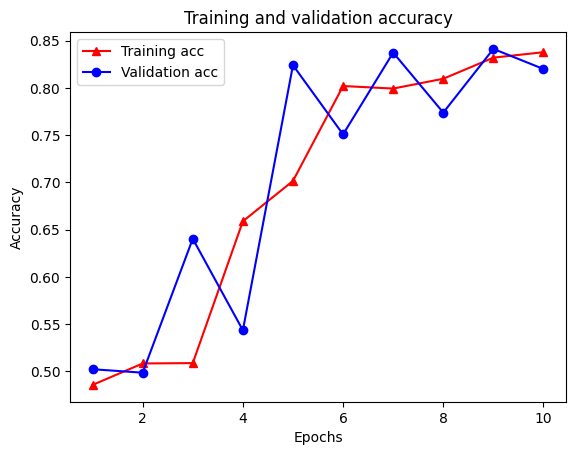## The power of semi-supervised learning

Semi-supervised learning and more specifically, graph regularization in the context of this tutorial, can be really powerful when the amount of training data is small. The lack of training data is compensated by leveraging similarity among the training samples, which is not possible in traditional supervised learning.

We define supervision ratio as the ratio of training samples to the total number of samples which includes training, validation, and test samples. In this notebook, we have used a supervision ratio of 0.05 (i.e, 5% of the labeled data) for training both the base model as well as the graph-regularized model. We illustrate the impact of the supervision ratio on model accuracy in the cell below.

``````# Accuracy values for both the Bi-LSTM model and the feed forward NN model have
# been precomputed for the following supervision ratios.

supervision_ratios = [0.3, 0.15, 0.05, 0.03, 0.02, 0.01, 0.005]

model_tags = ['Bi-LSTM model', 'Feed Forward NN model']
base_model_accs = [[84, 84, 83, 80, 65, 52, 50], [87, 86, 76, 74, 67, 52, 51]]
graph_reg_model_accs = [[84, 84, 83, 83, 65, 63, 50],
[87, 86, 80, 75, 67, 52, 50]]

plt.clf()  # clear figure

fig, axes = plt.subplots(1, 2)
fig.set_size_inches((12, 5))

for ax, model_tag, base_model_acc, graph_reg_model_acc in zip(
axes, model_tags, base_model_accs, graph_reg_model_accs):

# "-r^" is for solid red line with triangle markers.
ax.plot(base_model_acc, '-r^', label='Base model')
# "-gD" is for solid green line with diamond markers.
ax.plot(graph_reg_model_acc, '-gD', label='Graph-regularized model')
ax.set_title(model_tag)
ax.set_xlabel('Supervision ratio')
ax.set_ylabel('Accuracy(%)')
ax.set_ylim((25, 100))
ax.set_xticks(range(len(supervision_ratios)))
ax.set_xticklabels(supervision_ratios)
ax.legend(loc='best')

plt.show()
``````
`<Figure size 432x288 with 0 Axes>`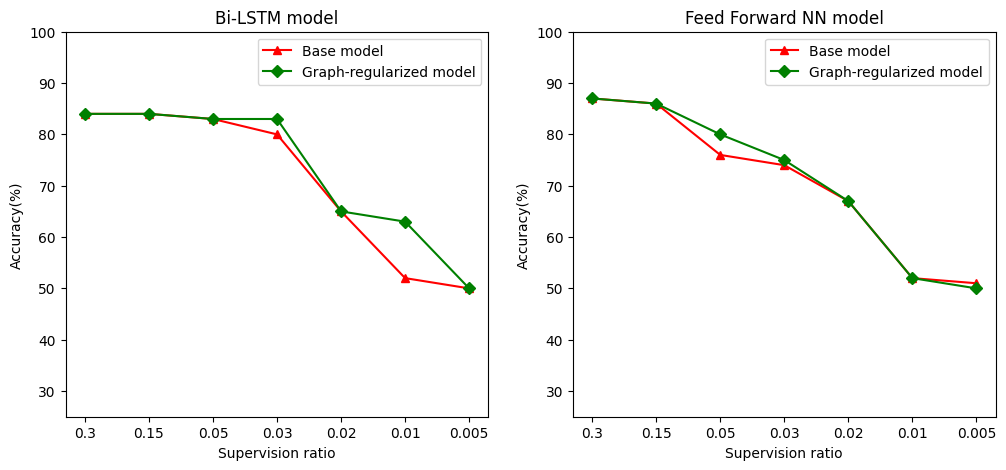It can be observed that as the superivision ratio decreases, model accuracy also decreases. This is true for both the base model and for the graph-regularized model, regardless of the model architecture used. However, notice that the graph-regularized model performs better than the base model for both the architectures. In particular, for the Bi-LSTM model, when the supervision ratio is 0.01, the accuracy of the graph-regularized model is ~20% higher than that of the base model. This is primarily because of semi-supervised learning for the graph-regularized model, where structural similarity among training samples is used in addition to the training samples themselves.

## Conclusion

We have demonstrated the use of graph regularization using the Neural Structured Learning (NSL) framework even when the input does not contain an explicit graph. We considered the task of sentiment classification of IMDB movie reviews for which we synthesized a similarity graph based on review embeddings. We encourage users to experiment further by varying hyperparameters, the amount of supervision, and by using different model architectures.